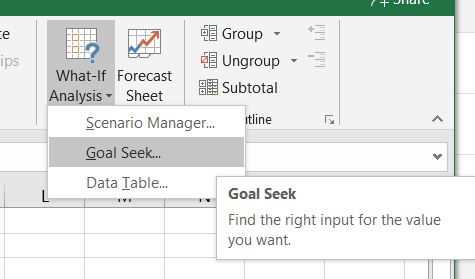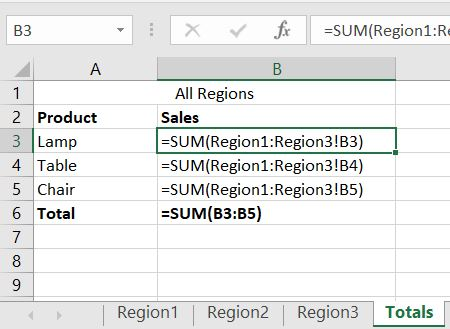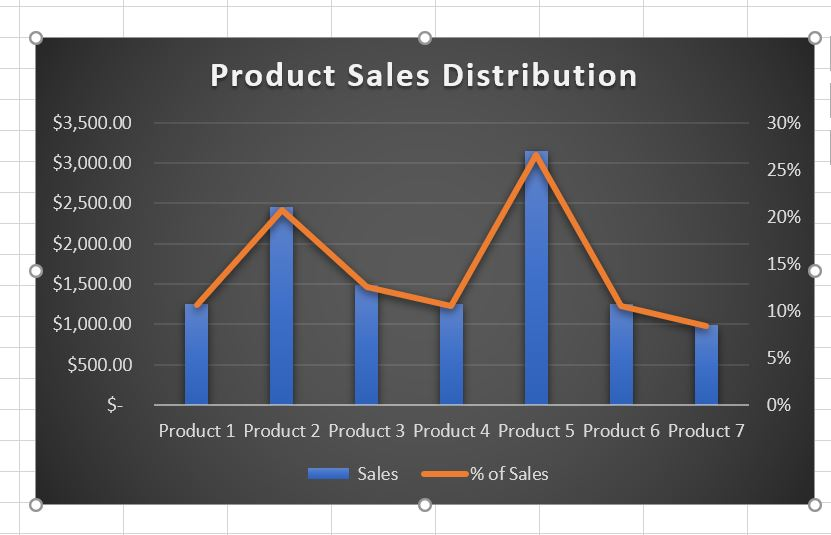Excel Reconciliation and Decimal PrecisionHow to reconcile Excel with a payroll system? I had a past student bring in a problem spreadsheet that they could not reconcile with their Quickbooks Payroll totals. This student had payroll data in an Excel spreadsheet and then entered employee data into Quickbooks to cut checks. At the end of every month the totals […]

How do you use Goal Seek in Excel?How do you use Goal Seek in Excel? Goal Seek in Excel is a seldom used tool that can help you find the number of units of a product you need to sell to break even for example.  It can also be used to find the proper price for a product to hit a desired […]

What is a Excel 3D Reference and How Do You Use One?What is a Excel 3D Reference and How Do You Use One? Excel 3D References can come in handy when you need to perform a calculation on data located in the same cell across multiple worksheets. These type of calculations are sometimes forgotten. There is also some confusion on how to set it up. Below […]

How to add a percentage column in a pivot table.Excel Pivot Tables are sometimes a mystery to users of Excel. In this example I am going to show you how to add a percentage (%) column into a pivot table. This column will calculate that rows percentage of the grand total. We will start with some sample raw data. In the worksheet above we […]

How to create a dual axis chart in ExcelCreating a dual axis chart In my opinion, charting in Excel is an art that needs to be practiced extensively to be an expert. As I demonstrate many examples in class I like to give additional info here for reference, for past, or future students. In this example I will show you how to create […]

How to add a call out Arrow to an Excel SpreadsheetHow to add a call out Arrow to an Excel Spreadsheet Lets pretend that you have an Excel Spreadsheet with an important number that you do not want your viewers to miss. How do you add a call out arrow to your spreadsheet? Below is our sample spreadsheet with a total under the last row […]

How to calculate age in ExcelHow do you calculate age using a formula or function in Excel To calculate age in Excel is relatively easy if you know the proper function to use. In this quick example I am going to use the today() function to perform some simple date math. In the below example we have a column of […]

Missing Row and Column Headings in ExcelExcel Tips and Helpful Info for StudentsExcel Information and Tips Excel is an extremely powerful program. Most users do not take advantage of all of the capabilities Excel has to offer. Below, please find some links to some of the more helpful Excel Tips I have found. Please note I did not create the articles below. I am just linking to […]

How to remove spaces in cell data in ExcelHow to remove spaces in cell data in Excel This came up in the last Excel class. A student asked how to remove spaces in Excel cell data. For example a product number that was entered incorrectly. In the below simple example we are going to use the SUBSTITUTE() fucntion in Excel to substitute a space […]